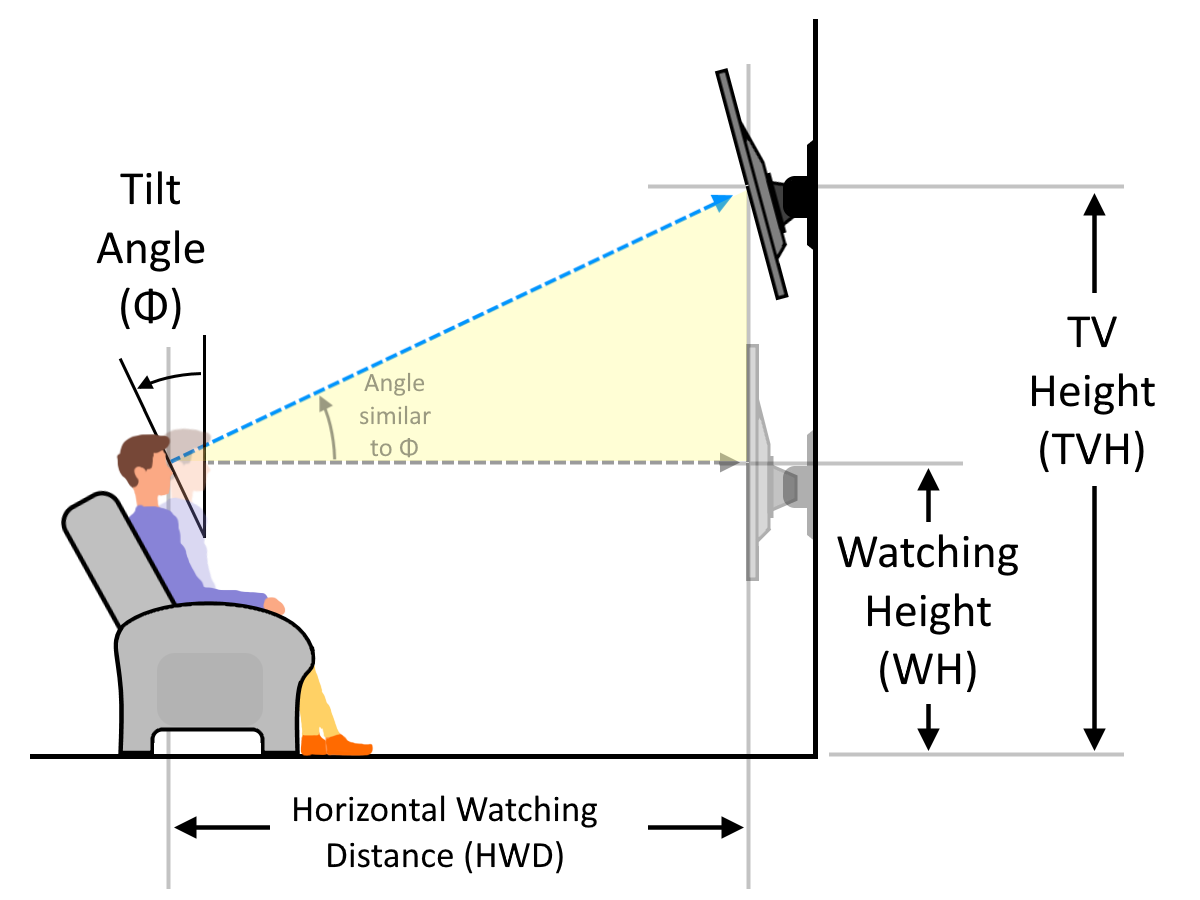If you need to know how high to mount a TV on the wall 📺 – this is our TV mounting height calculator that assist you find the ideal TV height. Not sure how high to mount a tv? If so, this is the perfect place for getting the answer. Take a second to review the photo above to familiarize yourself with the idea.

Regardless if you want to use to mount your TV in an office, in a bedroom, or a living room. Our tool will tell you the best height for the TV, considering the screen size and the tilt angle (also known as the reclining angle). Here we go!

## How high to mount a TV? Ideal TV height formula

There is an easy way to estimate how high a TV should be mounted. Let’s take a look at the optimal TV height formula:

TVH = WH + HWD * tan(Φ),

where:

• TVH is the ideal TV height;
• WH is the watching height – the level of the viewers’ eyes 👀;
• HWD is the horizontal watching distance 📏; and
• Φ is the tilt angle.

You can derive this equation by applying an appropriate trigonometric function for the yellow triangle (tan stands for the tangent function). Yup, here is the practical use of trigonometry in real life!

## How to find the best height for TV?

As we know how high to hang a TV, it’s reasonable to ask why the tv mounting height equation remains as it is.

One of the crucial factors is viewing height. Which honestly can be different for each person, use, and seating arrangement. It’s best to watch a screen when it’s at the same height as the viewer.  At least, when you are sitting straight in your chair or couch.

If your head tilts when sitting on the couch or the chair 🛋️, the ideal TV height, naturally, increases.

“Well how high should I mount my TV then?” – you may ask. Our TV mounting height calculator is here to help!

1. When the tilt angle is zero, the TV mount on the wall equals the watching height.
2. If the tilt angle is greater than zero (usually between 10° and 20°), then you can imagine three lines that form a right triangle 📐:
• The horizontal distance between the viewer and the TV;
• Vertical one from the eye-level to the center of the TV; and
• The one between your eyes and the TV’s center.

The ratio between vertical and horizontal lines equals the tangent of the tilt angle, so additional height above the eye-level is h = HWD * tan(Φ). Finally, the total height of the TV on the wall is the sum of these values, precisely the same as the ideal TV height formula from the previous section.

Maybe math isn’t for you? You could try the simpler method. Sit on the couch or chair in your normal viewing position. Now look at the wall the TV in going on. The center of your TV should be 15 degrees higher than your natural viewing angle.

## How to use our TV mounting height calculator?

Let’s see how high to mount a TV on a wall using our tool. Let’s assume you have a 65″ screen, and your eyes are around 40″ above the ground while sitting. The aspect ratio of your TV is a standard 16:9. The most comfortable position for you to watch is when the tilt angle equals 10°. So how high should a 65-inch tv be mounted?

1. Estimate the viewing distance. The ideal one for the viewing angle of 30° and a 65″ screen is 150.71″. You can always choose a different viewing angle in Advanced mode or simply type your viewing distance;
2. Work out the tangent of the tilt angle, tan(10°) = 0.1763. We round the value to four significant figures;
3. Apply the TV mounting height formula to find the best height for your TV: TVH = 105.71″ * 0.1763 + 40″ = 58.64″;
4. We can also convert the length to other units to 4.89 ft or 1.49 m.

Whenever we mention the optimal TV height, we mean its center. To evaluate the height of the TV’s bottom edge, we can continue our computations:

1. Using proportions and the screen diagonal‘s length, we can estimate the length of its vertical edge, 9 / √337 = x / 55″, so x = 9 * 65″ / √337 = 31.87″. The number within the square root is the following sum: 16² + 9² = 337.
2. The TV’s bottom edge is simply a half-length of the vertical one below the TV’s center, so 58.64″ – 31.87″/2 = 42.71″ is the height above the ground level.

As you can see, now you can work out all of the useful dimensions by yourself. However, if you don’t want to waste time, use our TV mounting height calculator, input a few measures, mount your TV at the ideal height, and enjoy the show 🍿📺🍹!

Wojciech Sas, PhD candidate